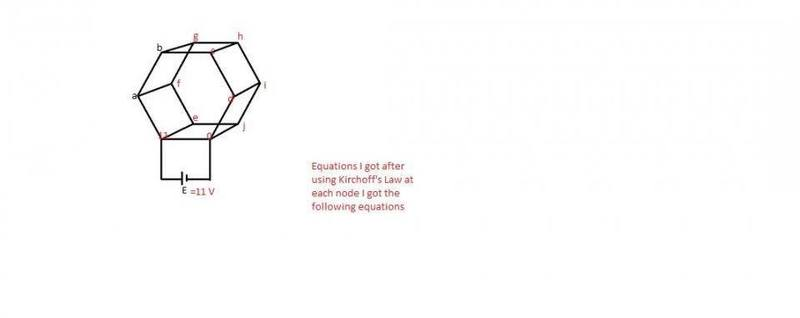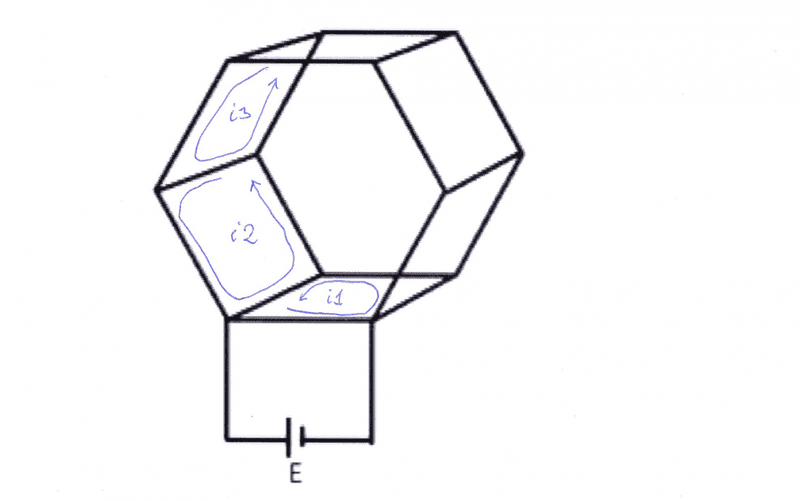# Hexagonal Grid Resistance

Sanchayan

## Answers and Replies

Gold Member
Why is Kirchhoff's voltage law (KVL) of no use?

You can setup 7 equations as for 7 loops ( 6 loops in the hexagonal itself and one loop including the battery). The current flowing through the battery is simply the loop-current through the battery.

Sanchayan
That will make it too complicated.I mean there must be a simpler way!! This problem was a 3 min MCQ question in my institute's previous year's question paper!

Gold Member
Well, you said that KVL is of no use, but that is not true: It is complicated.

Set R = 1Ω, calculate the current, then divide the result by 6. It then takes no time to setup the equations. But of course you spend some time to enter the equations in your calculator.

Maybe you can find some electrical symmetry in the circuit and cut it into pieces, but if you spend 10min to do so, I will have solved the problem before you with KVL.Sanchayan
Well ok,but how are you taking the potentials of the nodes?
I'm having problem in framing the equations P :-(!!

Gold Member
At first a suggestion: What will happen if you move the connections to the battery a ½R into the paper? ( middle of the ribs ). Then there is some symmetry.

Well ok,but how are you taking the potentials of the nodes?
You must draw the 7 loops with positive direction of circulation. Call the loop-currents i1 . . i7. Now follow exactly what kirchhoff states.
In the KVL, you must substitute ΔV by R*I. In this way you will find the currents ( not the voltages ).
Give it a try. Post your drawing and just one of the equations.

SanchayanI considered a potential for each node and then applied Kirchhoff 's current law at each junction I got about 10 equations with 10 variables.
For example for the node a : (a-11)/6+(a-b)/6+(a-f)/6=0 .

[Resistance of each side is 6 ohms]

But solving so many equations manually is really tough!!!!
@Hesch help now!!!

Gold Member
You are not drawing current-loops, but nodes.
Drawing (only 3 loops ):Equation as for i2:

-4*R*i2 + R*i3 + R*i1 = 0 , R = 1 =>

-4*i2 + i3 + i1 = 0

Now, try with other 6 loops.

Sanchayan
How did you write the equation for the loop involving the cell ?I got the rest of the 6 equations but I guess my cell equation is wrong!
@Hesch

Last edited:
Gold Member
You follow the arrow for i2: You will cross 4 resistors looping around, with the value R. therefore i2 results in a voltage drop = -4*R*i2.

One of the resistors in the loop is crossed by the current i3 in opposite direction, which yield a voltage rise = R*i3.

One other resistors is crossed by the current i1 in opposite direction, which yield a voltage rise = R*i1.

The sum of all these voltage changes must be zero, thus:

-4*R*i2 + R*i3 + R*i1 = 0.

Setting R = 1Ω, this can be rewritten:

-4*i2 + i3 + i1 = 0

Be very careful with the signs. ( That's why you have to make a drawing, so that you know what you are doing ). Having calculated the currents, remember to divide their values by 6, as the real value for R is 6Ω ( not 1Ω ).

Sanchayan
You follow the arrow for i2: You will cross 4 resistors looping around, with the value R. therefore i2 results in a voltage drop = -4*R*i2.

One of the resistors in the loop is crossed by the current i3 in opposite direction, which yield a voltage rise = R*i3.

One other resistors is crossed by the current i1 in opposite direction, which yield a voltage rise = R*i1.

The sum of all these voltage changes must be zero, thus:

-4*R*i2 + R*i3 + R*i1 = 0.

Setting R = 1Ω, this can be rewritten:

-4*i2 + i3 + i1 = 0

Be very careful with the signs. ( That's why you have to make a drawing, so that you know what you are doing ). Having calculated the currents, remember to divide their values by 6, as the real value for R is 6Ω ( not 1Ω ).

@Hesch I got the 6 equations as follows:
i2+i6=4*i1
i3+i1=4*i2
i2+i4=4*i3
i3+i5=4*i4
i4+i6=4*i5
i1+i5=4*i6

Then,the solution of these six equations is i1=i2=i3=i4=i5=i6=0 !!!
All the currents in all loops is coming as 0!!!
What does that mean??

Gold Member
Then,the solution of these six equations is i1=i2=i3=i4=i5=i6=0 !!!
Of course it will give 0: You have not connected the battery! One more loop including the battery is needed.
i2+i6=4*i1

You should write it as: i2 - 4*i4 + i6 = 0 because that's what Kirchhoff states: The sum of . . . . . . = 0. Only the voltage of a battery/powersupply, you will write on the right side. This is important because Kirchhoffs laws are intended for a computer, that can very systematically build the set of equation itself. Just "show" it at drawing/diagram, and it will do it.

You are the one to learn the computer to do it by programming. So do it systematically yourself, and you can learn the computer to do the same. Then it will solve 50 equations for you at the speed of light.

Last edited:
Sanchayan
Of course it will give 0: You have not connected the battery! One more loop including the battery is needed.

You should write it as: i2 - 4*i4 + i6 = 0 because that's what Kirchhoff states: The sum of . . . . . . = 0. Only the voltage of a battery/powersupply, you will write on the right side. This is important because Kirchhoffs laws are intended for a computer, that can very systematically build the set of equation itself. Just "show" it at drawing/diagram, and it will do it.

You are the one to learn the computer to do it by programming. So do it systematically yourself, and you can learn the computer to do the same. Then it will solve 50 equations for you at the speed of light.

How do i write the 7 th equation involving the battery?
@Hesch

Gold Member
With a clockwise loop:

i1 - i7 = -11V

Remember to include i7 in the loop for i1.

Sanchayan
With a clockwise loop:

i1 - i7 = -11V

Remember to include i7 in the loop for i1.

I'm getting current in cell as (15.46875/6)=2.57 (approx) am I right?

Gold Member
I'm getting current in cell as (15.46875/6)=2.57 (approx) am I right?
Well, 2.578125A ≈ 2.58A

So an important conclusion is: You can find currents with KVL, and you can find voltages by KCL.
It's very effective.

Sanchayan
Well, 2.578125A ≈ 2.58A

So an important conclusion is: You can find currents with KVL, and you can find voltages by KCL.
It's very effective.

Thanks a ton for the help @Hesch :-)!! Have a good day :-)!!Bbye :-)